Functions and CALL Routines

# MARGRCLPRC Function

Calculates call prices for European options on stocks, based on the Margrabe model.
 Category: Financial

## Syntax

 MARGRCLPRC(X1, t, X2, sigma1, sigma2, rho12)

### Arguments

X1

is a non-missing, positive value that specifies the price of the first asset.

 Requirement: Specify X1 and X2 in the same units.
t

is a non-missing value that specifies the time to expiration.

X2

is a non-missing, positive value that specifies the price of the second asset.

 Requirement: Specify X2 and X1 in the same units.
sigma1

is a non-missing, positive fraction that specifies the volatility of the first asset.

 Requirement: sigma1 must be for the same time period as the unit of t.
sigma2

is a non-missing, positive fraction that specifies the volatility of the second asset.

 Requirement: Specify a value for sigma2 for the same time period as the unit of t.
rho12

specifies the correlation between the first and second assets,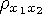.

 Range: between -1 and 1

The MARGRCLPRC function calculates the call price for European options on stocks, based on the Margrabe model. The function is based on the following relationship:
where

 X1 specifies the price of the first asset. X2 specifies the price of the second asset. N specifies the cumulative normal density function.
where

 t specifies the time to expiration.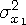specifies the variance of the first asset.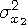specifies the variance of the second asset.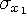specifies the volatility of the first asset.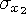specifies the volatility of the second asset.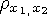specifies the correlation between the first and second assets.

For the special case of t=0, the following equation is true:
Note:   This function assumes that there are no dividends from the two assets.For information about the basics of pricing, see Using Pricing Functions.

The MARGRCLPRC function calculates the call price for European options on stocks, based on the Margrabe model. The MARGRPTPRC function calculates the put price for European options on stocks, based on the Margrabe model. These functions return a scalar value.

SAS Statements Results

`----+----1----+----2--`
```a=margrclprc(500, .5, 950, 4, 5, 1);
put a;```
`46.441283642`
```b=margrclprc(850, 1.2, 125, 5, 3, 1);
put b;```
`777.67008185`
```c=margrclprc(7500, .9, 950, 3, 2, 1);
put c;```
`6562.0354886`
```d=margrclprc(5000, -.5, 237, 3, 3, 1);
put d;```
`           0`

 Functions:Previous Page | Next Page | Top of Page